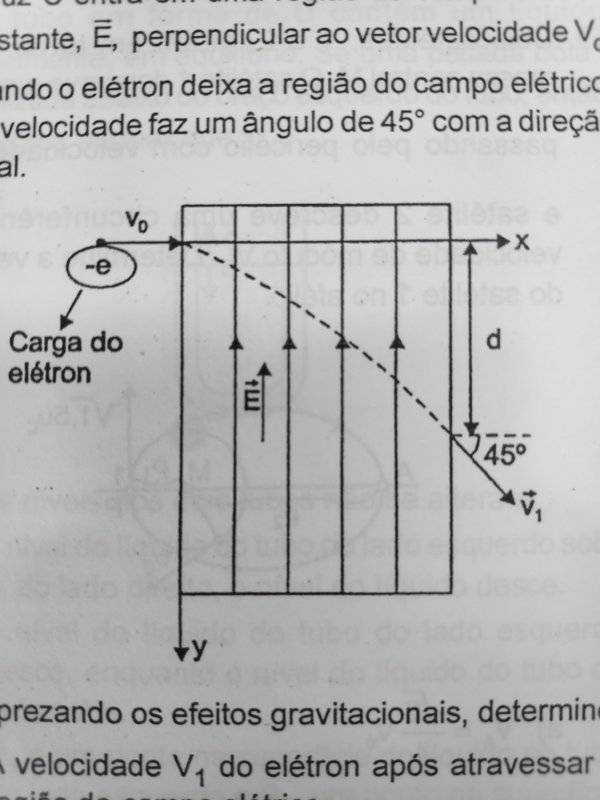# Electron moving inside a region of homogeneous electric field

Homework Statement:
An electron moving with velocity Vo=0,6C where C is light speed, enters in a region with uniform Electric Field, E, perpendicular to the vector Vo. When the electron leaves this region, it's velocity makes an angle of π/4 with it's inicial direction.
a) find velocity of electron after leaving the region with the electric Field.
b) find the distance d shown in the figure if E is the electric Field and Eo is the rest energy of the electron
Relevant Equations:
Etot^2=(pc)^2+Eo^2
U=Ed
W(electric)=Uea) since the eletric field is perpendicular to the inicial velocity, the x component is constant, hence Vf.cos45=Vo. This gives Vf=0,6√2.C
b) Ei=γi.Eo , γi=5/4 , Ef=γf.Eo , γf=5/(2√7)
Finally, Ei+e.E.d=Ef. Apparently this is incorrect, why??

PeroK
Homework Helper
Gold Member
2020 Award
Homework Statement:: An electron moving with velocity Vo=0,6C where C is light speed, enters in a region with uniform Electric Field, E, perpendicular to the vector Vo. When the electron leaves this region, it's velocity makes an angle of π/4 with it's inicial direction.
a) find velocity of electron after leaving the region with the electric Field.
b) find the distance d shown in the figure if E is the electric Field and Eo is the rest energy of the electron
Homework Equations:: Etot^2=(pc)^2+Eo^2
U=Ed
W(electric)=Ue

View attachment 253750
a) since the eletric field is perpendicular to the inicial velocity, the x component is constant, hence Vf.cos45=Vo. This gives Vf=0,6√2.C
b) Ei=γi.Eo , γi=5/4 , Ef=γf.Eo , γf=5/(2√7)
Finally, Ei+e.E.d=Ef. Apparently this is incorrect, why??

You have a two-dimensional relativistic scenario here. The acceleration is not in general parallel to the force.

•Moara
PeroK